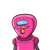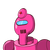# A cyclist goes around a circular track once every two minutes .if the radius of the circular track is 105 metres , calculate it’s

A cyclist goes around a circular track once every two minutes .if the radius of the circular track is 105 metres , calculate it’s speed??​

### 2 thoughts on “A cyclist goes around a circular track once every two minutes .if the radius of the circular track is 105 metres , calculate it’s”

1.If the radius of the circular track is 105 metre, calculate his speed. (Given pi= (22)/(7)) Step by step solution by experts to help you in doubt clearance & scoring excellent marks in exams. Thus, the speed of cyclist on the circular track is 5.5 metres per second.

2.Given,

A cyclist goes around a circular track once every two minutes .if the radius of the circular track is 105 metres , calculate it’s speed??

To find,

speed

Solution,

We know that for a body moving in a circular path :

$$v \: = \: \frac{2\pi \: r}{t} \\ speed \: = \: v \: = \: to \: be \: calculated \\ \pi = \frac{22}{7} \\ radius \: of \: track \: = \: r = 105m \\ time \: taken \: = 2min \\ 2 \times 60sec \\ 120sec \\ speed \: = v = \: \frac{2 \times 22 \times 105}{7 \times 120} \\ 5.5m \: per \: sec$$

The speed of cyclist on the circular track is 5.5 metres per second